Question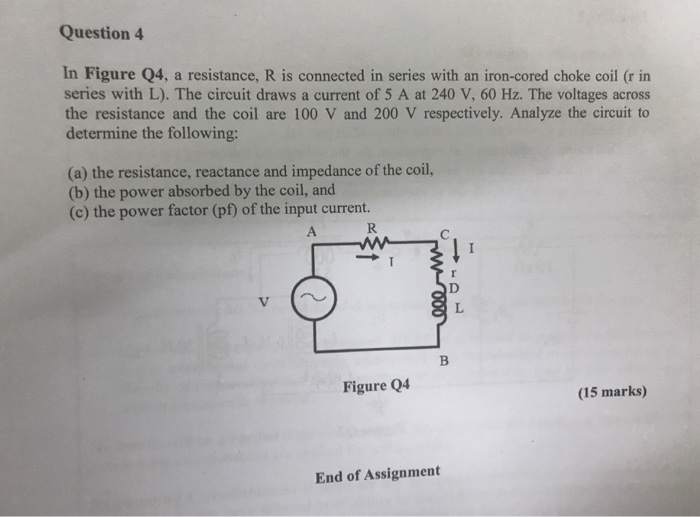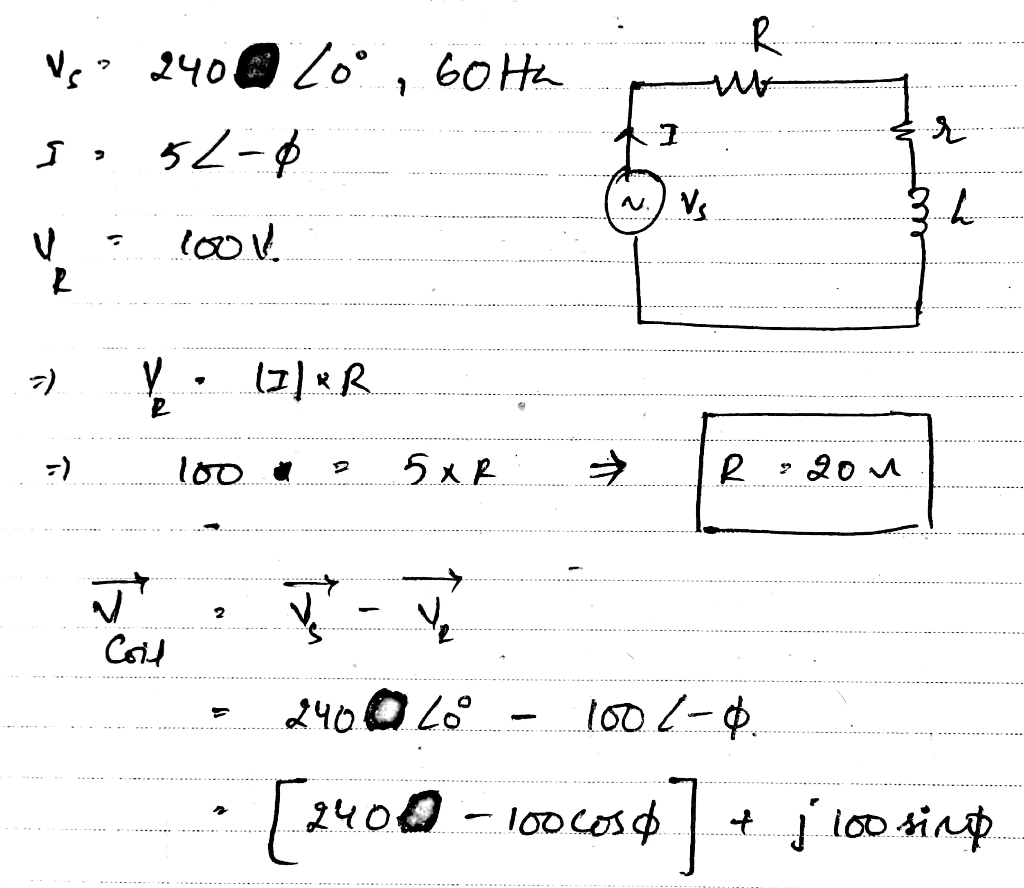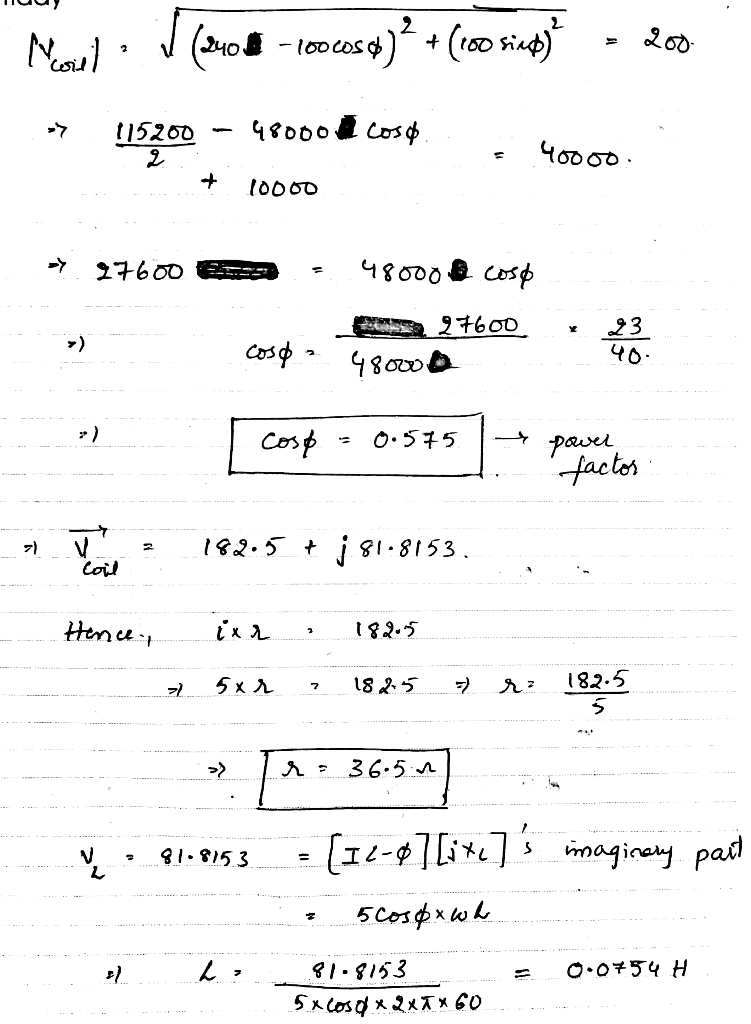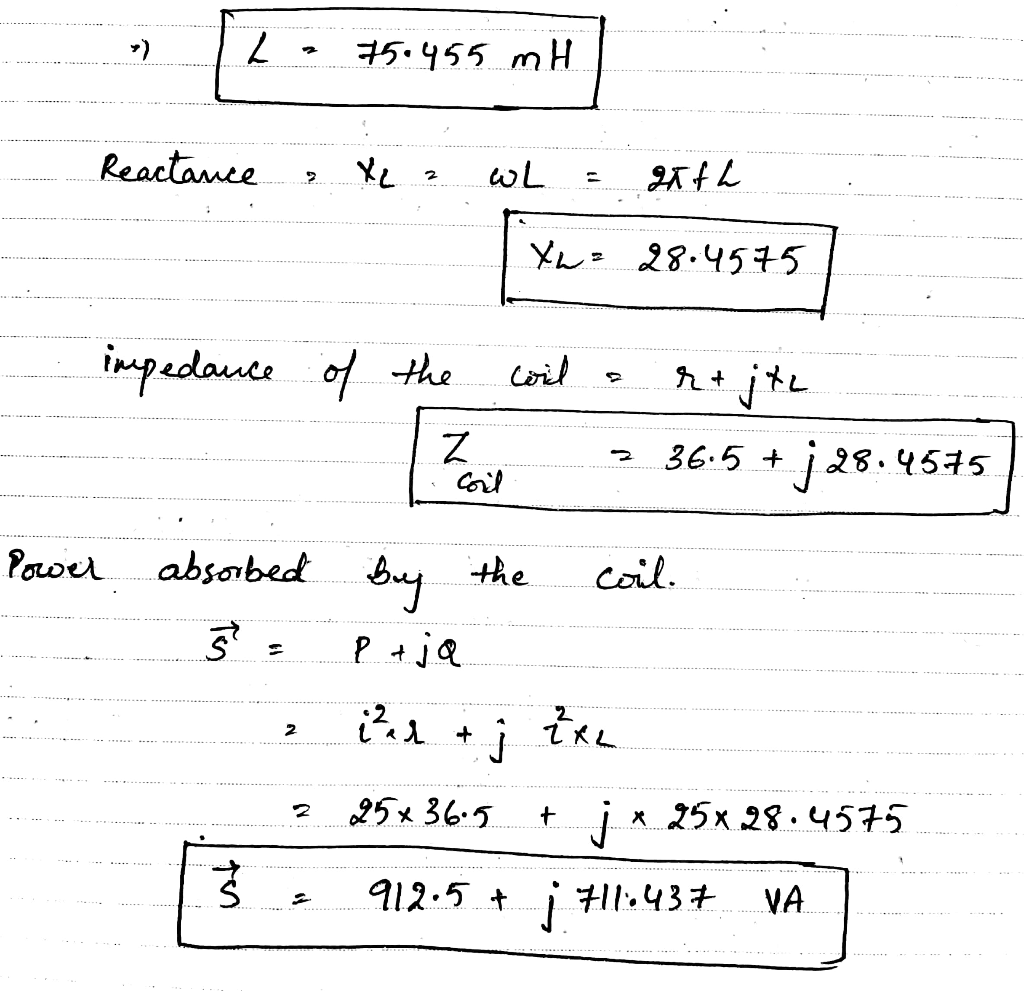#### Earn Coins

Coins can be redeemed for fabulous gifts.

Similar Homework Help Questions
• ### A large transformer operating at no-load draws an exciting current of 5 A when the primary is connected to a 240 V, 50 Hz source (HELP)

Question 1: A large transformer operating at no-load draws an exciting current  of 5 Awhen the primary is connected to a 240 V, 50 Hz source. From wattmeter testit is known that the iron losses are equal to 300 W. At short circuit the voltage applied is 24 Volts , the short-circuit current is 35 Amperes, the power meter reads 400 W. Calculate:i) The reactive power absorbed by the core;ii) The value of Rc  and Xm of the magnetising branch; iii)...

• ### An ac circuit with a 60 μF capacitor in series with a coil of resistance 18Ω...

An ac circuit with a 60 μF capacitor in series with a coil of resistance 18Ω and inductance 180mH is connected to a 100V, 100 Hz supply is shown below. Calculate inductive reactance                                                                           capacitive reactance                                                                          circuit impedance and phase angle θ circuit current I                                                                                          phasor voltages VR, VL, VC and VS                                                         resonance circuit frequency                                                                   construct a fully labeled voltage phasor diagram

• ### A single phase motor draws a current of 30 A from a 240 V, 60 Hz....

A single phase motor draws a current of 30 A from a 240 V, 60 Hz. line. Refer to Problem Description 7.2. A capacitor with a reactance of 31 Ω is then connected in parallel with the motor. A Wattmeter connected to the circuit gives a reading of 5.4 kW. How much reactive power does the capacitor deliver? How much net reactive power remains in the circuit? How much apparent power does the circuit now consume? What is the power...

• ### A 130 V (rms), 62 Hz power supply is connected to an RLC series circuit with...

A 130 V (rms), 62 Hz power supply is connected to an RLC series circuit with R = 145 Ω , L = 1.35 mH , and C = 31.0 μF . Part A: Find the reactance of the capacitor Part B: Find the reactance of the inductor Part C: Find the circuit impedance Part D: Find the peak current

• ### X=30R ㅔ Zoov 60HZ 32,- 4412 XL = 9052 R₂ = 3652. A Series Circuit Connected...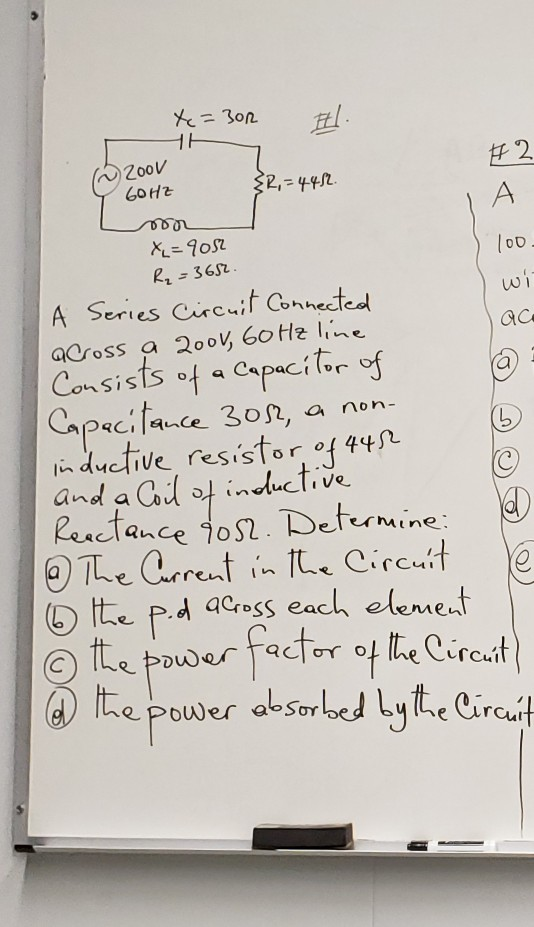X=30R ㅔ Zoov 60HZ 32,- 4412 XL = 9052 R₂ = 3652. A Series Circuit Connected across a 2001, 60 Hz line Consists of a Capacitor of Capacitance 30%, a non- inductive resistor of 445 and a Coil of inductive Reactance 9052. Determine: @ The Current in the Circuit 6 the p.d across each element © the power factor of the Circuit) @ the power absorbed by the Circuit

• ### X=30R ㅔ Zoov 60HZ 32,- 4412 XL = 9052 R₂ = 3652. A Series Circuit Connected...X=30R ㅔ Zoov 60HZ 32,- 4412 XL = 9052 R₂ = 3652. A Series Circuit Connected across a 2001, 60 Hz line Consists of a Capacitor of Capacitance 30%, a non- inductive resistor of 445 and a Coil of inductive Reactance 9052. Determine: @ The Current in the Circuit 6 the p.d across each element © the power factor of the Circuit) @ the power absorbed by the Circuit

• ### Circuit Analysis in Electrical Engineering 4. A resistor and a capacitor are connected in series across a 270-V ac supply. When the frequency is 40 Hz, the current flowing in the circuit is 6.25...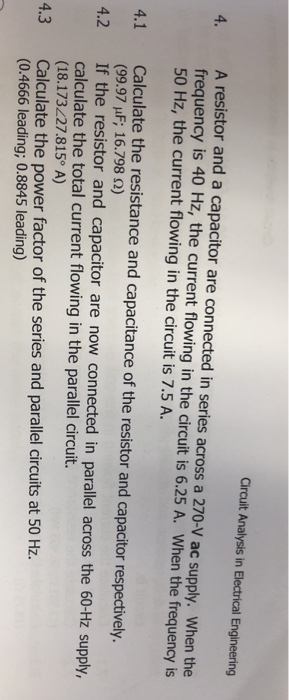Circuit Analysis in Electrical Engineering 4. A resistor and a capacitor are connected in series across a 270-V ac supply. When the frequency is 40 Hz, the current flowing in the circuit is 6.25 A. When the frequency is 50 Hz, the current flowing in the circuit is 7.5 A Calculate the resistance and capacitance of the resistor and capacitor respectively. (99.97 μF; 16.798 Ω) If the resistor and capacitor are now connected in parallel across the 60-Hz supply, calculate...

• ### 2. An AC power source with V 220 V and f60.0 Hz is connected in a...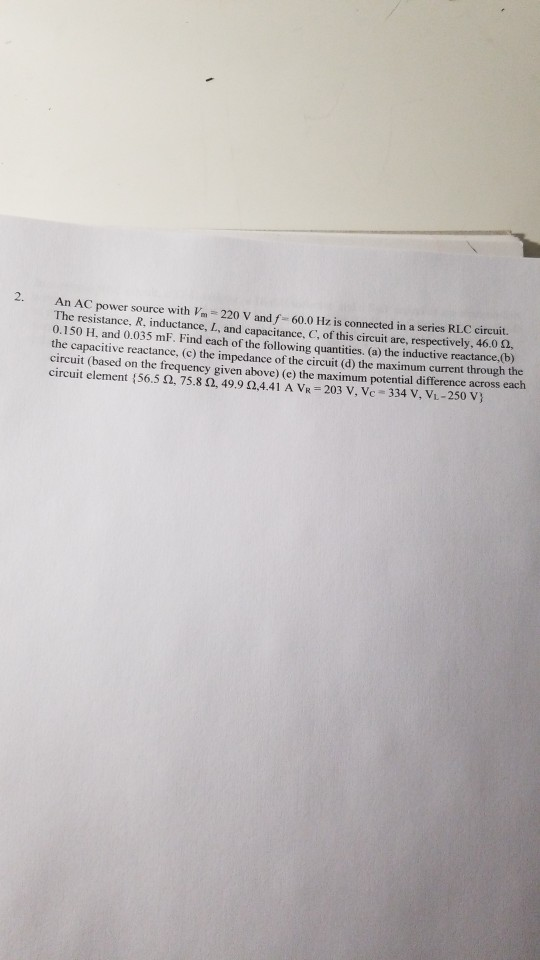2. An AC power source with V 220 V and f60.0 Hz is connected in a series RLC circuit. The resistance, R. inductance, L, and capacitance. C. of this circuit are, respectively. 46.0 12, 0.150 H. and 0.035 mF. Find each of the following quantities, (a) the inductive reactance, the capacitive reactance, (c) the impedance of the circuit (d) the maximum current through the circuit (based on the frequency given above) (e) the maximum potential difference across each circuit element...

• ### A series R-L-C circuit is connected to a 110 Hz ac source that has Vrms =...

A series R-L-C circuit is connected to a 110 Hz ac source that has Vrms = 80.0 V . The circuit has a resistance of 65.0 Ω and an impedance of 104 Ω at this frequency. What average power is delivered to the circuit by the source?

• ### Explore The RLC series circuit llustrated in the Active Figure has R-1.94 ?, L -1.87 H,...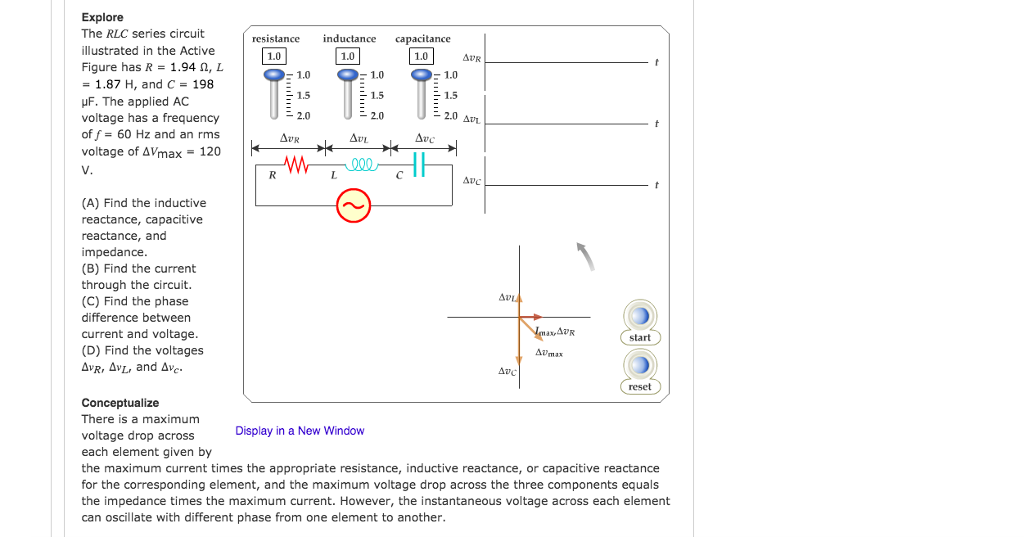Explore The RLC series circuit llustrated in the Active Figure has R-1.94 ?, L -1.87 H, and C 198 ?F. The applied AC voltage has a frequency of f- 60 Hz and an rms voltage of AVmax 120 resistance inductance capacitance 1.0 1.0 1.0 1.0 1.0 1,5 1.5 2.0 2.0 ?? AUR UC (A) Find the inductive reactance, capacitive reactance, and impedance (B) Find the current through the circuit. (C) Find the phase difference between current and voltage (D) Find...